# How to Calculate Retirement Date and Remaining Years refer to Birth Date in Excel

Every company has its own police about the retirement date for employees. Suppose employees will be retired from a certain age of 60, how can we calculate the retirement date for everyone base on their birthdays? Actually, there are several functions can calculate the retirement date in excel, you can use them in proper formula, then we will get the correct result. This article will provide you some useful functions.

For example. We have a list of employee’s information. In this table, we can see the employees’ ID, name, birth date. And base on the birth date, we want to know their retirement date and remaining years. Now let’s follow below steps to find the solution.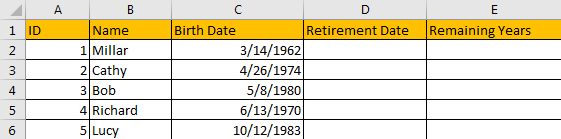## Calculate Retirement Date refer to Birth Date in Excel

Suppose you will be retired at age of 60-year-old. If you were born in 3/14/1985, you will be retired at 3/14/2045. You can add 60 on you birth year. As there are many employees need to be calculated in a company, so we need a simple formula to auto calculate the retirement date.

Step1: In D2 which shows the retirement date, enter the formula =EDATE(C2,12*60).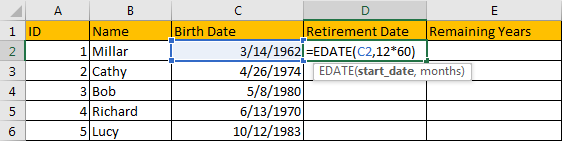In this formula, EDATE function can return a date which has the same date with the entered start date but comes in the future after several certain months. As the retirement year is 60, so parameter for months is 12*60 in this case.

Step 2: Click Enter to get the result. Verify we get a five-digits number. That’s because the returned number is General format.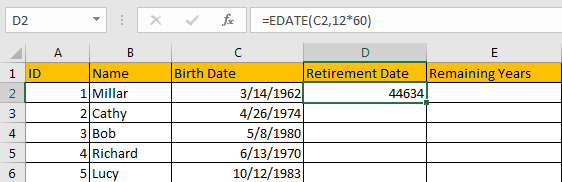Step 3: Select D2, click Home, in Number panel, click dropdown list (the default load value is General). Select Short Date.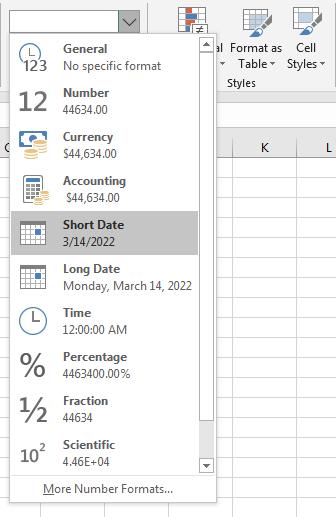Step 4: Verify that D2 is updated to short date format properly.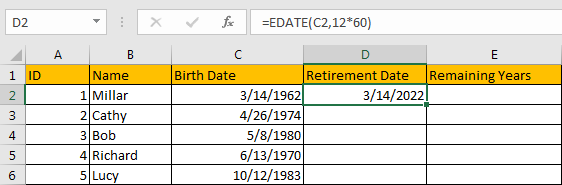Step 5: Drag the fill handler to fill D3-D6. Verify that retirement date is calculated properly in this column.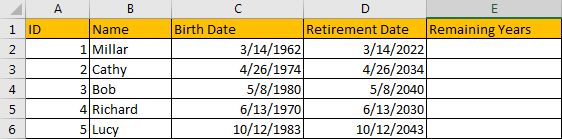## Calculate Remaining Years in Excel

Except the retirement date, we also want to know the remaining years before the retirement date.

Step 1: In E2, enter the formula =YEARFRAC(TODAY(),D2).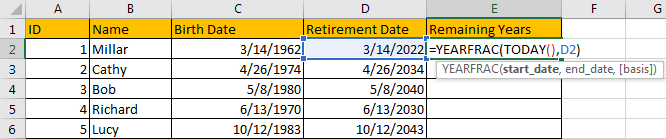In this formula, YEARFRAC Function calculates the ratio of days (take the number of full days) between two dates (start_date and end_date) to one year.

Step2: Click Enter to get the result. Verify that left year is calculated properly.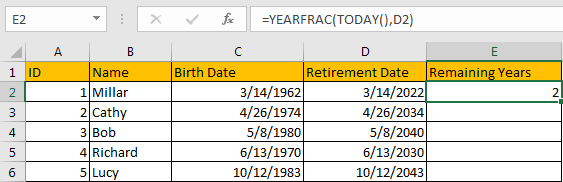Step 3: Drag the fill handler to fill E3-E6. Verify that remaining years is calculated properly in this column. Verify that remaining years is not always an integer due to dates between today and retirement date don’t equal to entire years.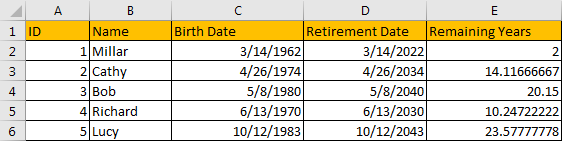Step 4: If you want to just keep the integer part without rounding, you can use INT function. For example in E2, edit formula =INT(YEARFRAC(TODAY(),D3)). Verify that 14 is displayed.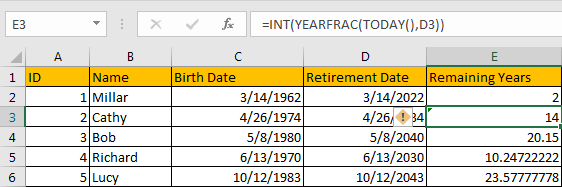### Related Functions

• Excel EDATE function
TThe Excel EDATE function returns the serial number that represents the date that is a specified number of months before or after a specified date.The syntax of the EDATE function is as below:=EDATE (start_date, months)…
• Excel TODAY function
The Excel TODAY function returns the serial number of the current date. So you can get the current system date from the TODAY function. The syntax of the TODAY function is as below:=TODAY()…
• Excel MONTH Function
The Excel MONTH function returns the month of a date represented by a serial number. And the month is an integer number from 1 to 12.The syntax of the MONTH function is as below:=MONTH (serial_number)…
• Excel INT function
The Excel INT function returns the integer portion of a given number. And it will rounds a given number down to the nearest integer.The syntax of the INT function is as below:= INT (number)…

Sidebar